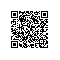# while语句

python中的while语句和其他语言没有什么不一样，我使用while语句来编写一个斐波拉赫数列：

In : while x < 10 :
...:     print(x)
...:     x, y = y, x+y
...:
0
1
1
2
3
5
8

# if 语句

python中的 if 可以和 elif 或者 else 配合使用：

>>> x = int(input("Please enter an integer: "))
Please enter an integer: 42
>>> if x < 0:
...     x = 0
...     print('Negative changed to zero')
... elif x == 0:
...     print('Zero')
... elif x == 1:
...     print('Single')
... else:
...     print('More')
...
More

if语句很简单，这里就不做过多的介绍。

# for语句

Python中的for语句主要用来对序列进行迭代，比如列表或者字符串：

In :  ages = [ 10, 14, 18, 20 ,25]
In : for age in ages:
...:     print(age)
...:
10
14
18
20
25

In : for age in ages.copy():
...:     print(age)
...:
10
14
18
20
25

for语句和range()函数的结合，可以得到不一样的效果。

range()用来生成给定范围内的集合：

In : for age in range(5):
...:     print(age)
...:
0
1
2
3
4

range()函数还可以带步长作为第三个参数：

In : for age in range(5, 10 , 2):
...:     print(age)
...:
5
7
9

Range()和len()组合，可以方便的变量列表：

>>> a = ['Mary', 'had', 'a', 'little', 'lamb']
>>> for i in range(len(a)):
...     print(i, a[i])
...
0 Mary
1 had
2 a
3 little
4 lamb

# Break

break用来跳出最近的for或者while循环。

In : for n in range(2, 10):
...:     for x in range(2, n):
...:         if n % x == 0:
...:             print(n, 'equals', x, '*', n//x)
...:             break
...:     else:
...:         print(n, 'is a prime number')
...:
2 is a prime number
3 is a prime number
4 equals 2 * 2
5 is a prime number
6 equals 2 * 3
7 is a prime number
8 equals 2 * 4
9 equals 3 * 3

# Continue

continue用来跳过此次循环中的后面部分，继续执行下一次循环。

In : for n in range(2, 10):
...:     for x in range(2, n):
...:         if n % x == 0:
...:               print(n, 'equals', x, '*', n//x)
...:               continue
...:     else:
...:         print(n, 'is a prime number')
...:
2 is a prime number
3 is a prime number
4 equals 2 * 2
4 is a prime number
5 is a prime number
6 equals 2 * 3
6 equals 3 * 2
6 is a prime number
7 is a prime number
8 equals 2 * 4
8 equals 4 * 2
8 is a prime number
9 equals 3 * 3
9 is a prime number

# pass

pass表示的是什么都不做。是一个空的执行。

>>> while True:
...     pass  # Busy-wait for keyboard interrupt (Ctrl+C)
...

>>> class MyEmptyClass:
...     pass
...

>>> def initlog(*args):
...     pass   # Remember to implement this!
...